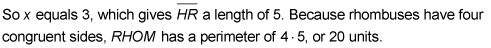##### Geometry Essentials For DummiesThe three special parallelograms — rhombus, rectangle, and square — are so-called because they’re special cases of the parallelogram. (In addition, the square is a special case or type of both the rectangle and the rhombus.)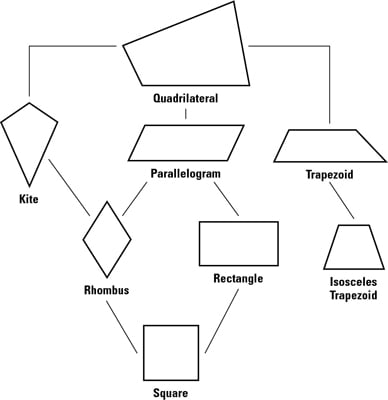The three-level hierarchy you see with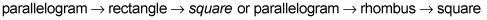in the above quadrilateral family tree works just like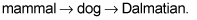A dog is a special type of a mammal, and a Dalmatian is a special type of a dog.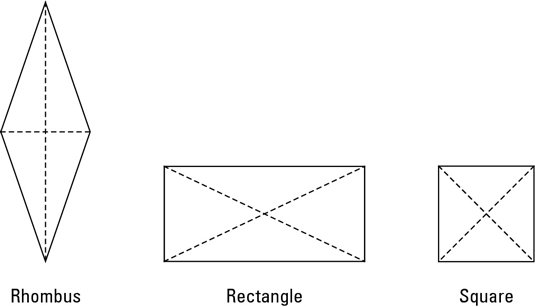Here are the properties of the rhombus, rectangle, and square. Note that because these three quadrilaterals are all parallelograms, their properties include the parallelogram properties.

• The rhombus has the following properties:

• All of the properties of a parallelogram apply (the ones that matter here are parallel sides, opposite angles are congruent, and consecutive angles are supplementary).

• All sides are congruent by definition.

• The diagonals bisect the angles.

• The diagonals are perpendicular bisectors of each other.

• The rectangle has the following properties:

• All of the properties of a parallelogram apply (the ones that matter here are parallel sides, opposite sides are congruent, and diagonals bisect each other).

• All angles are right angles by definition.

• The diagonals are congruent.

• The square has the following properties:

• All of the properties of a rhombus apply (the ones that matter here are parallel sides, diagonals are perpendicular bisectors of each other, and diagonals bisect the angles).

• All of the properties of a rectangle apply (the only one that matters here is diagonals are congruent).

• All sides are congruent by definition.

• All angles are right angles by definition.

Now try working through a problem. Given the rectangle as shown, find the measures of angle 1 and angle 2: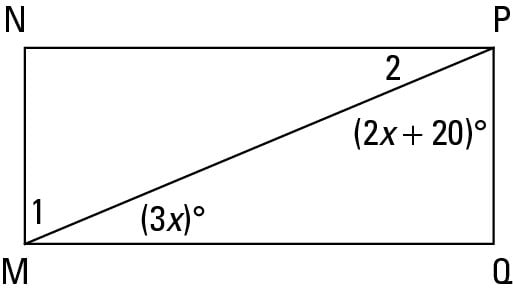Here’s the solution: MNPQ is a rectangle, so angle Q = 90°. Thus, because there are 180° in a triangle, you can say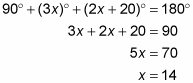Now plug in 14 for all the x’s.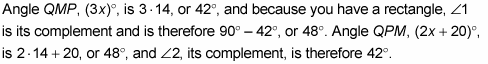Now find the perimeter of rhombus RHOM.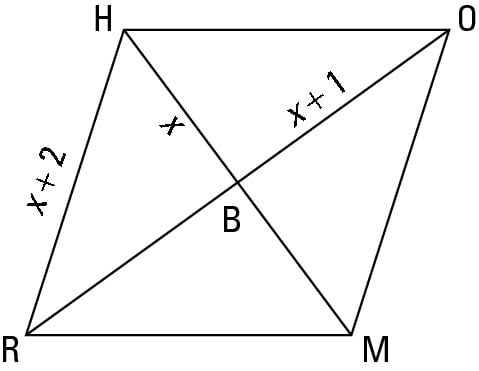Here’s the solution: All the sides of a rhombus are congruent, so HO equals x + 2. And because the diagonals of a rhombus are perpendicular, triangle HBO is a right triangle. You finish with the Pythagorean Theorem: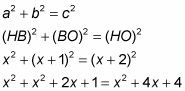Combine like terms and set equal to zero: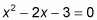Factor:

(x – 3)(x + 1) = 0

Use Zero Product Property:

x – 3 = 0 or x + 1 = 0

x = 3 or x = –1

You can reject x = –1 because that would result in triangle HBO having legs with lengths of –1 and 0.